# Extract or Filter exclude blank values

Let’s suppose that you have assigned a task in which you need to filter out the values by excluding the blank values from the list having few values, and I am also pretty sure about it that you would definitely choose to do it manually, which is also a great choice when you have only a few values in a list, and you want to filter them out by excluding the blank values.

But if you are dealing with multiple values in the list and you want to filter them out by excluding all the blank cells, then in such a situation doing these tasks manually would be a foolish act because doing it manually, there are 95% chances that you would 100% get bored of it and can’t complete your task at the right time.

But you don’t need to worry about it because after carefully reading this article filtering out the blank values from the list containing multiple values would become very easy for you.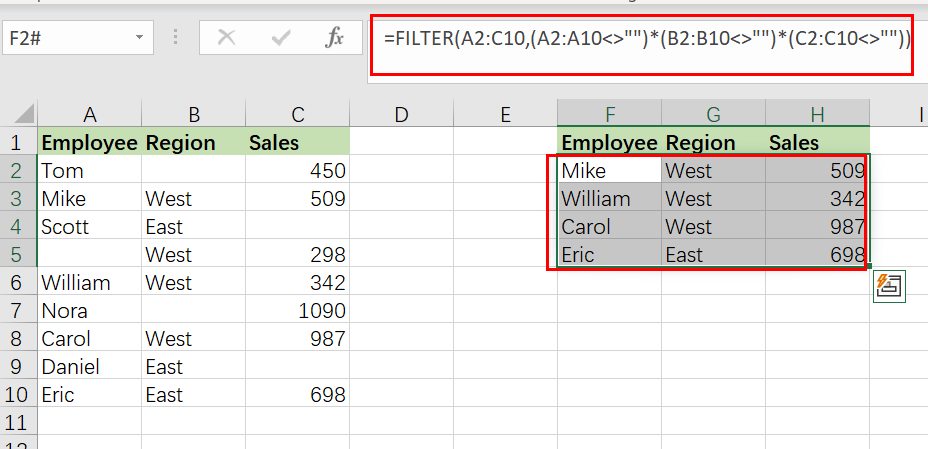So let’s go deep into the article to take you out of this problem.

## General Formula

The Following formula would help you to Filter exclude the blank values in MS Excel:

`=FILTER(total_data,(range1<>"")*( range2<>"")*( range3<>"")`

## Syntax Explanations

Before explaining the formula for getting the work done efficiently, we must understand each syntax, which would make it easy for us that how each syntax contributes to filtering out the top n values in MS Excel.

• `Filter`: This function contributes to narrowing down or filtering out a range of data on the user-defined criteria.
• `Comma symbol (,)`: In Excel, this comma symbol acts as a separator that helps to separate a list of values.
• `RangeN`: is nothing but representing each column from the list.
• `Parenthesis` (): The core purpose of this Parenthesis symbol is to group the elements and separate them from the rest of the elements.
• `total_data`: In your worksheet, it represents the input ranges.

## Let’s See How This Formula Works

For instance, you got a task in which there is a table where you have candidates of two regions (i.e., West and East ) and which are assigned to a particular sales number, now you want to filter out the values by excluding all the blank cells, now let’s analyze that how to to write the formula and how this formula would do it.

As to filter exclude blank values, we would write the formula according to the given list like:

`=FILTER(A2:C10,(A2:A10<>"")*(B2:B10<>"")*(C2:C10<>""))`The result from this formula would be a list of all data without any blank cell in between them.

The FILTER function extracts data that fits one or more criteria. In this scenario, we wish to use criteria that need data in all three source data columns.It means that if any of these values are missing from a row, that row should be exclude from the last result.

So in order to achieve the result, we have to use three boolean expressions that operate on arrays in Filter formula. The first expression checks for empty employee names:

`A2:A10<>"" // check employee names`

The “not equal” `operator (<>)` used with an empty string (“”) means “not blank values”. The result for each cell in the range A2:A10 will be TRUE or FALSE, where TRUE indicates “not empty” and FALSE means “empty.” Because the range has 9 cells, we obtain 9 results in an array-like as follows:

`={TRUE;TRUE;TRUE;FALSE;TRUE;TRUE;TRUE;TRUE;TRUE}`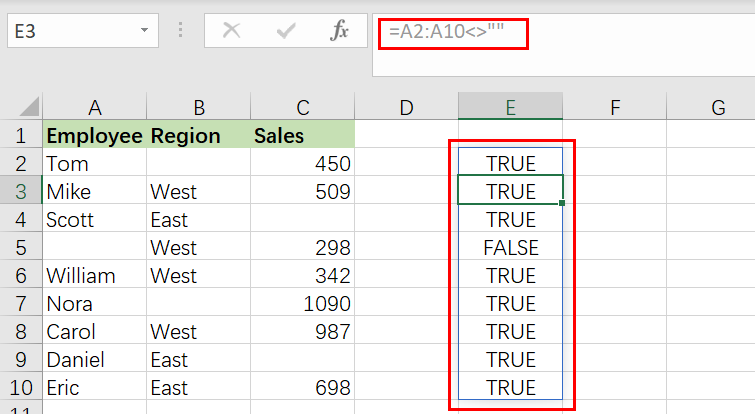The 2nd expression test for blank regions is as follows:

`B2:B10<>"" / check regions`

the result is as below:

`={FALSE;TRUE;TRUE;TRUE;TRUE;FALSE;TRUE;TRUE;TRUE}`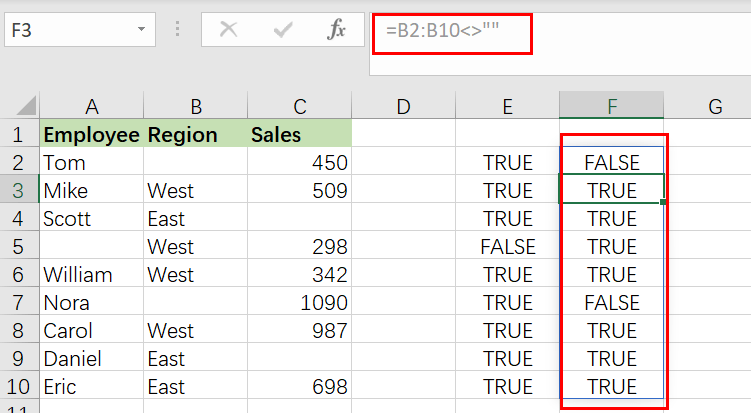Last, we look for empty sale numbers:

`C2:C10<>"" / check Sales`

which results in:

`={TRUE;TRUE;FALSE;TRUE;TRUE;TRUE;TRUE;FALSE;TRUE}`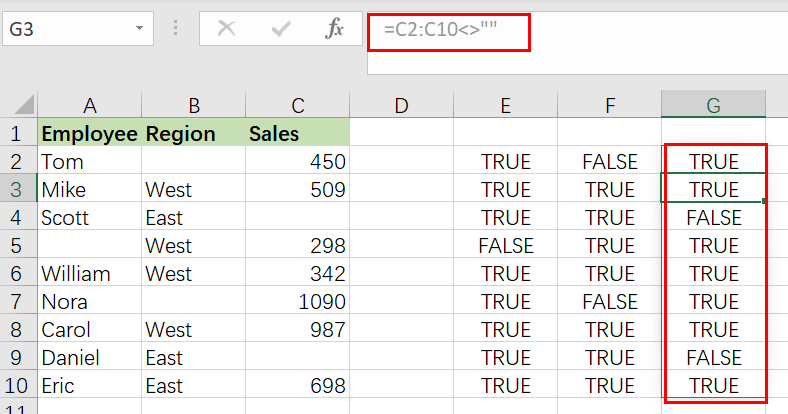`=(A2:A10<>"")*(B2:B10<>"")*(C2:C10<>"")`

When the three formulas above are multiplied, the arithmetic process converts the `TRUE` and `FALSE` values to` 1` and `0`. In this situation, we employ multiplication to enforce “`AND`” logic: expression1 AND expression2 AND expression3. In other words, in a given row, all three expressions must return True.

The end outcome, according to the laws of boolean logic, is an array like this:

`={0;1;0;0;1;0;1;0;1}`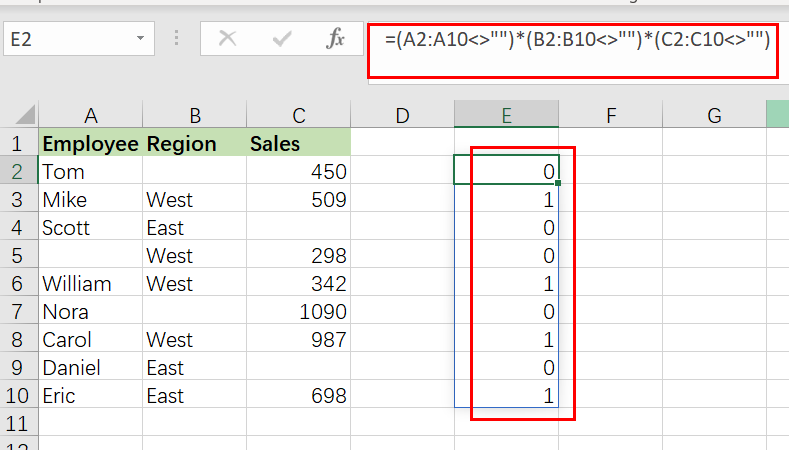This array is passed straight to the FILTER function as the include parameter to filter out all data excluding rows that have blank values.

### Related Functions

• Excel Filter function
The Excel  FILTER function extracts matched records from a collection of data using one or more logical checks. The include argument specifies logical tests, which might encompass a wide variety of formula conditions.==FILTER(array,include,[if empty])…